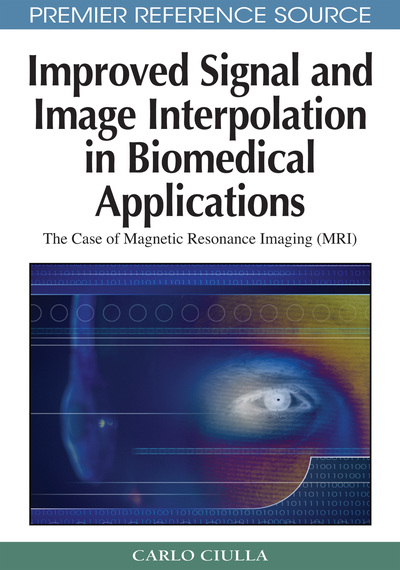# The Notion

Carlo Ciulla (Lane College, USA)
DOI: 10.4018/978-1-60566-202-2.ch006
Available
\$37.50
No Current Special Offers

## Abstract

The goal of this chapter is to discuss the practical implications of the notion. This chapter concludes the logical reasoning outlined through the process of deduction that begun in Chapter II while reporting on the intuition. The logical reasoning was necessary in order to arrive to a determination as to what is the meaning of the intuition. Along the way it was possible to introduce the novel conception of Intensity-Curvature Functional and to connect such conception to the initial intuition of SRE. At that point the intuition was assessed in its truth and thus it was manifest to reconsider the formulation of the SRE. The implication of doing so rests in that the formulation and the conceptualization of the SRE given in Chapter IV is useful for the remainder of the book in presenting the unifying theory for the improvement of the interpolation error. The process of deduction (logical reasoning) has finally arrived to the notion that the curvature of the model interpolation function is quite relevant to the extent of improving the approximation properties of the function. The realization of the notion was already anticipated in Chapter V and this chapter further extends on the notion through the illustrations of its practical implications. In other words the process of deduction has arrived to a beneficial conclusion and for this reason it proves itself to have been useful in order to derive the notion.
Chapter Preview
Top

## The Curvature Of The Interpolation Function

This chapter concludes Section I of the book deriving the notion from the evaluation of the truth foreseen in the intuition. The presentation is consequential to what already seen in the previous four chapters and it is particularly focused on the trivariate liner interpolation function.

The conception of the Intensity-Curvature Functional that was given in Chapter III has shown that it possible to give a mathematical formulation that is such to measure the energy level of the signal (image) before and after interpolation. The Intensity-Curvature Functional is thus capable to determine the energy level change of the signal (image) when is subject to re-sampling at any intra-voxel location through the model interpolation function. Based on this conception it was derived the empirical formulation of the Sub-pixel Efficacy Region (SRE), which deduction is subsequent to the study of the Intensity-Curvature Functional. Such formulation asserts that the Sub-pixel Efficacy Region is that spatial set of intra-voxel points where the energy level change of the signal (image) is minimum or maximum. This descends from the fact that the set of points that belong to the SRE are derived through the solution of the polynomial system of first order partial derivatives of the Intensity-Curvature Functional (ΔE) and that is the task that corresponds to the identification of the extremes of ΔE.

In Chapter II however, the definition given to the SRE was purely theoretical and was derived from the intuition. Definition VII seen in Chapter II was given within the context of the hyperbolic paraboloid function h = h(X, Y, Z) that exists for each (X, Y, Z) of the voxel Ψ and that is capable to estimate the signal at any intra-voxel location. The Sub-pixel Efficacy Region in Ψ was thus defined as that intra-voxel set of points:

Ω = { (X, Y, Z): | ∂2h /δxδy |z=Z = | ∂2h /δyδx |z=Z ≤ Z θxy and| ∂2h /δxδz |y=Y = | ∂2h /δzδx |y=Y ≤ Y θxz and| ∂2h /δyδz |x=X = | ∂2h /δzδy |x=X ≤ X θyz }.

Also, for convenience to the reader, it is herein recalled from definition V of Chapter II that:

2h /δxδy = ∂2h /∂y∂x = [f(0,0,0) – f(1,0,0) – f(0,1,0) + f(1,1,0)] + Z ωf2h/∂x∂z = ∂2h/∂z∂x = [f(0,0,0) – f(1,0,0) – f(0,0,1) + f(1,0,1)] + Y ωf2h /∂y∂z = ∂2h /∂z∂y = [f(0,0,0) – f(0,1,0) – f(0,0,1) + f(0,1,1)] + X ωf

## Complete Chapter List

Search this Book:
Reset